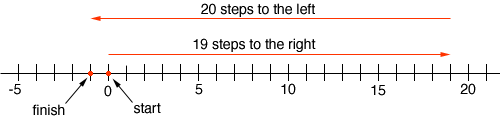Quandaries and Queries Who is asking: Student Level: Middle Question: NEED HELP : 46 + -24 = -38 + -22 = 19 - 20 = Hi Billy, Think about starting at zero and taking a number of steps along the number line where a positive number means you step to the right and a negative number means you step to the left. In your third problem you take 19 steps to the right then 20 steps to the left.You finish at -1 so 19 - 20 = -1 Now try the second problem. If you start at zero and take 38 steps to the left then a further 22 steps to the left where do you finish? Now try problem 1. Penny